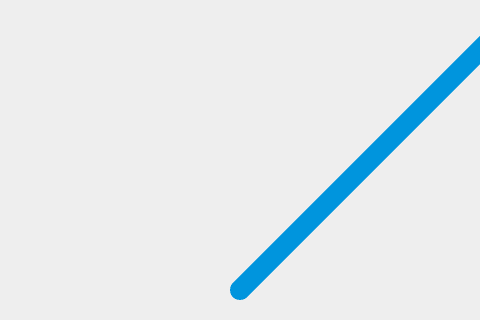# Move the ball

This is the 2nd step out of 10 of the Gamedev Canvas tutorial (en-US). You can find the source code as it should look after completing this lesson at Gamedev-Canvas-workshop/lesson2.html.

Você já sabe como desenhar uma bola através do tópico anterior, então agora vamos seguir em frente. Tecnicamente, nos vamos estar pintando uma bola na tela, clareando e então pintando de novo em uma posição um pouco diferente a cada frame para dar a impressão de movimento — assim como os filmes se movimentam.

## Defining a drawing loop

To keep constantly updating the canvas drawing on each frame, we need to define a drawing function that will run over and over again, with a different set of variable values each time to change sprite positions, etc. You can run a function over and over again using a JavaScript timing function such as `setInterval()` (en-US) or `requestAnimationFrame()`.

Delete all the JavaScript you currently have inside your HTML file except for the first two lines, and add the following below them. The `draw()` function will be executed within `setInterval` every 10 miliseconds:

``````function draw() {
// drawing code
}
setInterval(draw, 10);
``````

Thanks to the infinite nature of `setInterval` the `draw()` function will be called every 10 milliseconds forever, or until we stop it. Now, let's draw the ball — add the following inside your `draw()` function:

``````ctx.beginPath();
ctx.arc(50, 50, 10, 0, Math.PI*2);
ctx.fillStyle = "#0095DD";
ctx.fill();
ctx.closePath();
``````

Try your updated code now — the ball should be repainted on every frame.

## Making it move

You won't notice the ball being repainted constantly at the moment, as it's not moving. Let's change that. First, instead of a hardcoded position at (50,50) we will define a starting point at the bottom center part of the Canvas in variables called `x` and `y`, then use those to define the position the circle is drawn at.

First, add the following two lines above your `draw()` function, to define `x` and `y`:

``````var x = canvas.width/2;
var y = canvas.height-30;
``````

Next update the `draw()` function to use the x and y variables in the `arc()` method, as shown in the following highlighted line:

``````function draw() {
ctx.beginPath();
ctx.arc(x, y, 10, 0, Math.PI*2);
ctx.fillStyle = "#0095DD";
ctx.fill();
ctx.closePath();
}
``````

Now comes the important part: we want to add a small value to `x` and `y` after every frame has been drawn to make it appear that the ball is moving. Let's define these small values as `dx` and `dy` and set their values to 2 and -2 respectively. Add the following below your x and y variable definitions:

``````var dx = 2;
var dy = -2;
``````

The last thing to do is to update `x` and `y` with our `dx` and `dy` variable on every frame, so the ball will be painted in the new position on every update. Add the following two new lines indicated below to your `draw()` function:

``````function draw() {
ctx.beginPath();
ctx.arc(x, y, 10, 0, Math.PI*2);
ctx.fillStyle = "#0095DD";
ctx.fill();
ctx.closePath();
x += dx;
y += dy;
}
``````

Save your code again and try it in your browser. This works ok, although it appears that the ball is leaving a trail behind it:## Clearing the canvas before each frame

The ball is leaving a trail because we're painting a new circle on every frame without removing the previous one. Don't worry, because there's a method to clear canvas content: `clearRect()`. This method takes four parameters: the x and y coordinates of the top left corner of a rectangle, and the x and y coordinates of the bottom right corner of a rectangle. The whole area covered by this rectangle will be cleared of any content previously painted there.

Add the following highlighted new line to the `draw()` function:

``````function draw() {
ctx.clearRect(0, 0, canvas.width, canvas.height);
ctx.beginPath();
ctx.arc(x, y, 10, 0, Math.PI*2);
ctx.fillStyle = "#0095DD";
ctx.fill();
ctx.closePath();
x += dx;
y += dy;
}
``````

Save your code and try again, and this time you'll see the ball move without a trail. Every 10 milliseconds the canvas is cleared, the blue circle (our ball) will be drawn on a given position and the `x` and `y` values will be updated for the next frame.

## Cleaning up our code

We will be adding more and more commands to the `draw()` function in the next few articles, so it's good to keep it as simple and clean as possible. Let's start by moving the ball drawing code to a separate function.

Replace the existing draw() function with the following two functions:

``````function drawBall() {
ctx.beginPath();
ctx.arc(x, y, 10, 0, Math.PI*2);
ctx.fillStyle = "#0095DD";
ctx.fill();
ctx.closePath();
}

function draw() {
ctx.clearRect(0, 0, canvas.width, canvas.height);
drawBall();
x += dx;
y += dy;
}
``````